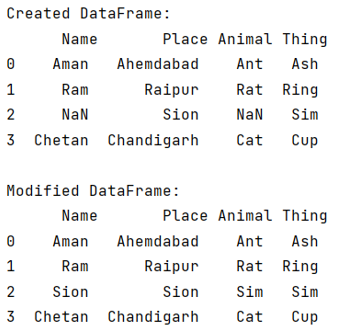# How to pass another entire column as argument to pandas fillna()?

Given a Pandas DataFrame, we have to pass another entire column as argument to pandas fillna(). By Pranit Sharma Last updated : September 23, 2023

Pandas is a special tool that allows us to perform complex manipulations of data effectively and efficiently. Inside pandas, we mainly deal with a dataset in the form of DataFrame. DataFrames are 2-dimensional data structures in pandas. DataFrames consist of rows, columns, and data.

## Problem statement

Suppose we have a DataFrame that consists of some Nan values or missing values, and we want to fill those values with the corresponding values of the adjacent column

## Passing another entire column as argument to pandas fillna()

For this purpose, we will use pandas.DataFrame.fillna() method and we will pass the other column as an argument in this method. The fillna() method fills NA/NaN values using the specified method.

Note

To work with pandas, we need to import pandas package first, below is the syntax:

```import pandas as pd
```

Let us understand with the help of an example,

## Python program to to pass another entire column as argument to pandas fillna()

```# Importing pandas package
import pandas as pd

# Importing numpy package
import numpy as np

# Creating a DataFrame
df = pd.DataFrame({
'Name':['Aman','Ram',np.NaN,'Chetan'],
'Animal':['Ant','Rat','Snake','Cat'],
'Thing':['Ash','Ring','Sim','Cup']
})

# Display DataFrame
print("Created DataFrame:\n",df,"\n")

# Replacing Nan with the values of other column
df['Name'] = df['Name'].fillna(df['Place'])
df['Animal'] = df['Animal'].fillna(df['Thing'])

# Display modified DataFrame
print("Modified DataFrame:\n",df)
```

### Output

The output of the above program is: